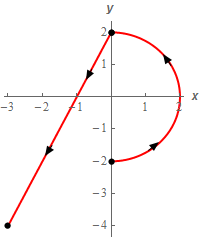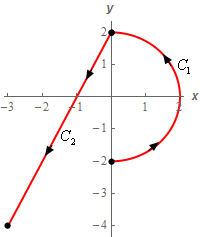Paul's Online Notes
Home / Calculus III / Line Integrals / Line Integrals - Part II
Show Mobile Notice Show All Notes Hide All Notes
Mobile Notice
You appear to be on a device with a "narrow" screen width (i.e. you are probably on a mobile phone). Due to the nature of the mathematics on this site it is best views in landscape mode. If your device is not in landscape mode many of the equations will run off the side of your device (should be able to scroll to see them) and some of the menu items will be cut off due to the narrow screen width.

### Section 16.3 : Line Integrals - Part II

4. Evaluate $$\displaystyle \int\limits_{C}{{1 + {x^3}\,dx}}$$ where $$C$$ is the right half of the circle of radius 2 with counter clockwise rotation followed by the line segment from $$\left( {0,2} \right)$$ to $$\left( { - 3, - 4} \right)$$. See the sketch below for the direction.Show All Steps Hide All Steps

Start Solution

To help with the problem let’s label each of the curves as follows,Now let’s parameterize each of these curves.

$${C_1}:\,\,\vec r\left( t \right) = \left\langle {2\cos \left( t \right),2\sin \left( t \right)} \right\rangle \hspace{0.25in} - \frac{1}{2}\pi \le t \le \frac{1}{2}\pi$$
$${C_2}:\,\,\vec r\left( t \right) = \left( {1 - t} \right)\left\langle {0,2} \right\rangle + t\left\langle { - 3, - 4} \right\rangle = \left\langle { - 3t,2 - 6t} \right\rangle \hspace{0.25in}0 \le t \le 1$$

Show Step 2

Now we need to compute the line integral for each of the curves.

\begin{align*}\int\limits_{{{C_1}}}{{1 + {x^3}\,dx}} & = \int_{{ - \,\,\frac{1}{2}\pi }}^{{\frac{1}{2}\pi }}{{\left[ {1 + {{\left( {2\cos \left( t \right)} \right)}^3}} \right]\left( { - 2\sin \left( t \right)} \right)\,dt}}\\ & = \int_{{ - \,\,\frac{1}{2}\pi }}^{{\frac{1}{2}\pi }}{{ - 2\sin \left( t \right) - 16{{\cos }^3}\left( t \right)\sin \left( t \right)\,dt}}\\ & = \left. {\left( {2\cos \left( t \right) + 4{{\cos }^4}\left( t \right)} \right)} \right|_{ - \,\frac{1}{2}\pi }^{\frac{1}{2}\pi } = \underline 0 \end{align*} \begin{align*}\int\limits_{{{C_2}}}{{1 + {x^3}\,dx}} & = \int_{0}^{1}{{\left[ {1 + {{\left( { - 3t} \right)}^3}} \right]\left( { - 3} \right)\,dt}}\\ & = \int_{0}^{1}{{ - 3 + 81{t^3}\,dt}}\\ & = \left. {\left( { - 3t + \frac{{81}}{4}{t^4}} \right)} \right|_0^1 = \underline {\frac{{69}}{4}} \end{align*}

Don’t forget to correctly deal with the differentials when converting the line integral into a “standard” integral.

Show Step 3

Okay to finish this problem out all we need to do is add up the line integrals over these curves to get the full line integral.

$\displaystyle \int\limits_{C}{{1 + {x^3}\,dx}} = \left( 0 \right) + \left( {\frac{{69}}{4}} \right) = \require{bbox} \bbox[2pt,border:1px solid black]{{\frac{{69}}{4}}}$

Note that we put parenthesis around the result of each individual line integral simply to illustrate where it came from and they aren’t needed in general of course.